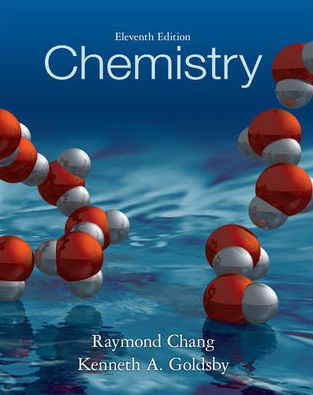×
Get Full Access to Chemistry - 11 Edition - Chapter 7 - Problem 75p
Get Full Access to Chemistry - 11 Edition - Chapter 7 - Problem 75p

×

# Answer: Indicate which of the following sets of quantumISBN: 9780073402680 118

## Solution for problem 75P Chapter 7

Chemistry | 11th Edition

• Textbook Solutions
• 2901 Step-by-step solutions solved by professors and subject experts
• Get 24/7 help from StudySoup virtual teaching assistantsChemistry | 11th Edition

4 5 1 280 Reviews
18
5
Problem 75P

Indicate which of the following sets of quantum numbers in an atom are unacceptable and explain why:

(a) $$\left(1,\ 0,\ \frac{1}{2},\ \frac{1}{2}\right)$$, (b) $$\left(3,\ 0,\ 0,\ +\frac{1}{2}\right)$$, (c) $$\left(2,\ 2,\ 1,\ +\frac{1}{2}\right)$$, (d) $$\left(4,\ 3,\ -2,\ +\frac{1}{2}\right)$$, (e) $$(3,\ 2,\ 1,\ 1)$$.

Step-by-Step Solution:

Step 1 of 6

Here we have to indicate which of the following sets of quantum numbers in an atom are unacceptable and explain why:

The given set of quantum numbers are,

(a) (1, 0, ½ , ½ ) (b) (3, 0 , 0, + ½ ) (c) (2, 2, 1, + ½ ) (d) (4, 3, -2, + ½ ) (e) (3, 2, 1, 1)

It is known that the quantum numbers can be calculated as follows,

Principal quantum number (n):- It is the quantum number which indicates the number of shell i.e 1,2,3,4... etc.

Azimuthal quantum number (l) :- The value of azimuthal quantum number for different orbitals are as follows,

Orbital   s p d f

Value     0 1 2 3….(0 to n-1)

Magnetic quantum number(ml) :- Magnetic quantum can be calculated as ml = -l to +l

Spin quantum number (ms) value will be always +½ or -½

Step 2 of 6

Step 3 of 6

##### ISBN: 9780073402680

The full step-by-step solution to problem: 75P from chapter: 7 was answered by , our top Chemistry solution expert on 11/08/17, 03:59AM. Since the solution to 75P from 7 chapter was answered, more than 367 students have viewed the full step-by-step answer. The answer to “?Indicate which of the following sets of quantum numbers in an atom are unacceptable and explain why: (a) $$\left(1,\ 0,\ \frac{1}{2},\ \frac{1}{2}\right)$$, (b) $$\left(3,\ 0,\ 0,\ +\frac{1}{2}\right)$$, (c) $$\left(2,\ 2,\ 1,\ +\frac{1}{2}\right)$$, (d) $$\left(4,\ 3,\ -2,\ +\frac{1}{2}\right)$$, (e) $$(3,\ 2,\ 1,\ 1)$$.” is broken down into a number of easy to follow steps, and 42 words. Chemistry was written by and is associated to the ISBN: 9780073402680. This full solution covers the following key subjects: atom, explain, indicate, Numbers, quantum. This expansive textbook survival guide covers 25 chapters, and 3591 solutions. This textbook survival guide was created for the textbook: Chemistry, edition: 11.

## Discover and learn what students are asking

Chemistry: The Central Science : Molecular Geometry and Bonding Theories
?What is the distinction between a bond dipole and a molecular dipole moment?

Unlock Textbook Solution

Enter your email below to unlock your verified solution to:

Answer: Indicate which of the following sets of quantum